# Graph Neural Networks for Fraud Detection in Crypto Transactions – Towards AI#### Author(s): Maria Zorkaltseva

Originally published on Towards AI the World’s Leading AI and Technology News and Media Company. If you are building an AI-related product or service, we invite you to consider becoming an AI sponsor. At Towards AI, we help scale AI and technology startups. Let us help you unleash your technology to the masses.

In this tutorial, we will apply Graph Convolutional Network (GCN) and Graph Attention Network (GAT) to detect fraudulent bitcoin transactions. Also, we will compare their performances.Photo by DeepMind on Unsplash

#### Table of Contents

Introduction
Spectral-based Convolutional GNN
Attention-based spatial Convolutional GNN
Dataset
Node classification with GCN/GAT using PyTorch Geometric (PyG)
References

#### Introduction

Despite significant progress within deep learning areas such as computer vision, natural language/audio processing, time series forecasting, etc., the majority of problems work with non-euclidian geometric data and as an example of such data are social network connections, IoT sensors topology, molecules, gene expression data and so on. The non-Euclidian nature of data implies that all properties of Euclidian vector space R^n can not be applied to such data samples; for example, shift-invariance, which is an important property for Convolutional Neural Networks (CNN), does not save her. In  the authors explain how convolution operation can be translated to the non-Euclidian domain using spectral convolution representation for graph structures. At present, Graph Neural Networks (GNN) have found their application in many areas:

physics (particle systems simulation, robotics, object trajectory prediction)
chemistry and biology (drug and protein interaction, protein interface prediction, cancer subtype detection, molecular fingerprints, chemical reaction prediction)
combinatorial optimizations (used to solve NP-hard problems such as traveling salesman problem, minimum spanning trees)
traffic networks (traffic prediction, taxi demand problem)
recommendation systems (links prediction between users and content items, social network recommendations)
computer vision (scene graph generation, point clouds classification, action recognition, semantic segmentation, few-shot image classification, visual reasoning)
natural language processing (text classification, sequence labeling, neural machine translation, relation extraction, question answering)

Among the classes of state-of-the-art GNNs, we can distinguish them into recurrent GNNs, convolutional GNNs, graph autoencoders, generative GNNs, and spatial-temporal GNNs.

In this tutorial, we will consider the semi-supervised node classification problems using Graph Convolutional Network and Graph Attention Network and compare their performances on the Elliptic dataset, which contains crypto transaction data. Also, we will highlight their building block concepts, which come from spectral-based and spatial-based representations of convolution.

#### Spectral-based Convolutional GNN

Spectral-based models take their mathematical basis from the graph signal processing field; among known models are ChebNet, GCN, AGCN, and DGCN. To understand the principle of such models, let’s consider the concept of spectral convolution [2, 3].

Let’s say we have a graph signal x from R^n, which is the feature vector of all nodes of a graph, and x_i is a value of a i-th node. This graph signal is first transformed to the spectral domain by applying Fourier transform to conduct a convolution operator. After the convolution, the resulting signal is transformed back using the inverse graph Fourier transform. These transforms are defined as: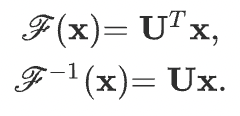Here U is the matrix of eigenvectors of the normalized graph Laplacian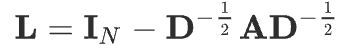where D is the degree matrix, A is the adjacency matrix of the graph, and I_N is the identity matrix. The normalized graph Laplacian can be factorized as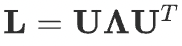Based on the convolution theorem, the convolution operation with filter g can be defined as: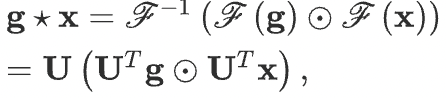if we denote a filter as g as a learnable diagonal matrix of U^T*g, then we get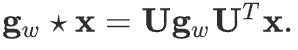We can understand g_w as a function of the eigenvalues of L. Evaluation of multiplication with the eigenvector matrix U takes O(N²) time complexity; to overcome this problem, in ChebNet and GCN, Chebyshev polynomials are used. For ChebNet, spectral convolution operation is represented as follows.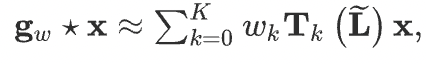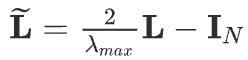To circumvent the problem of overfitting, in GCN, Chebyshev approximation with K=1 and lambda_max = 2 is used. And convolutional operator will become as follows.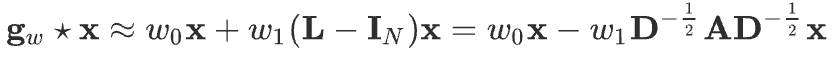Assuming, w = w_0 = -w_1, we get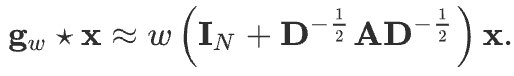GCN further introduces a normalization trick to solve the exploding/vanishing gradient problem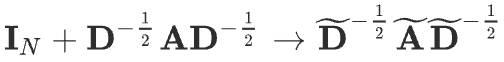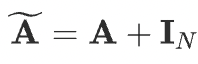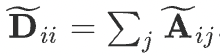Finally, the compact form of GCN is defined as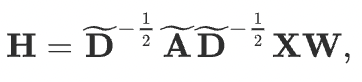Here X is the input feature matrix, dim(X) = N x F^0, N is the number of nodes, and F^0 number of input features for each node;

A is the adjacency matrix, dim(A) = N x N;

W is the weights matrix, dim(W) = F x F’, F is the number of input features, F’ is the number of output features;

H represents a hidden layer of graph neural network, dim(H) = N x F’.

At each i-th layer H_i, features are aggregated to form the next layer’s features, using the propagation rule f (e.g. sigmoid/relu), and thus features become increasingly abstract at each consecutive layer, which reminds the principle of CNN.

#### Attention-based spatial Convolutional GNN

Among spatial-based convolutional GNN models, the following models are widely known: GraphSage, GAT, MoNet, GAAN, DiffPool, and others. The working principle is similar to CNN convolution operator application to image data, except the spatial approach applies convolution to differently sized node neighbors of a graph.

Attention mechanism gained wide popularity thanks to the models used in NLP tasks (e.g., LSTM with attention, transformers). In the case of GNN having an attention mechanism, contributions of neighboring nodes to the considered node are neither identical nor pre-defined, as, for example, in GraphSage or GCN models.

Let’s look at the attention mechanism of GAT ; normalized attention coefficients for this model can be calculated via the following formula: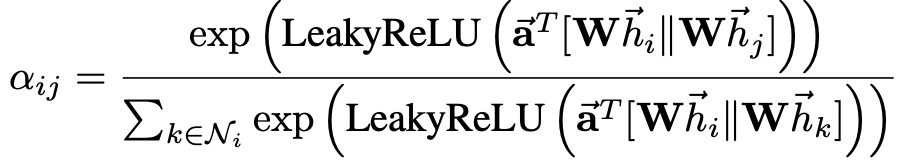Here, T represents transposition and || is concatenation operation;

h is a set of node features (N is a number of nodes, F is a number of features in each node)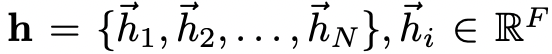W is weight matrix (linear transformation to a features), dim(W) = F’ x F

Vector a is the weight vector for a single-layer feed-forward neural network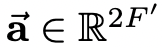The softmax function ensures that the attention weights sum up to one overall neighbour of the i-th node.

Finally, these normalized attention coefficients are used to compute a linear combination of the features corresponding to them, to serve as the final output features for every node.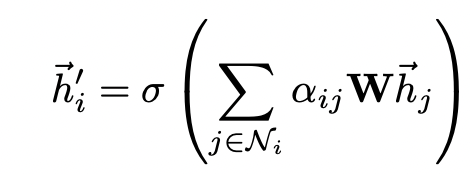Usage of single self-attention can lead to instabilities, and in this case, multi-head attention with K independent attention mechanisms is used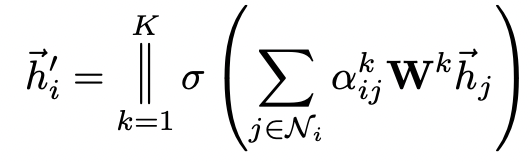#### Dataset

Here, for the node classification task, we will use the Elliptic dataset. Dataset consists of 203 769 nodes and 234 355 edges. There are three categories of nodes: “licit”, “illicit”, or “unknown”. A node is deemed “licit” / “illicit” if the corresponding transaction has been created by an entity that belongs to a licit (exchanges, wallet providers, miners, financial service providers, etc.) or illicit (scams, malware, terrorist organizations, ransomware, Ponzi schemes, etc.) category respectively. A detailed description of that dataset is available in the following article, “The Elliptic Data Set: opening up machine learning on the blockchain”.

#### Node classification with GCN/GAT using PyTorch Geometric (PyG)

Here we will consider a semi-supervised node classification problem using PyG library, where nodes will be transactions and edges will be transactions flows.

https://medium.com/media/16b39837dd095fdff88384a73f01be17/href

You can simply import the Elliptic bitcoin dataset from PyG pre-installed datasets using the instructions down below, but for the sake of clarity, let’s build PyG dataset object by ourselves. Raw data can be downloaded via this link.

from torch_geometric.datasets import EllipticBitcoinDataset
dataset = EllipticBitcoinDataset(root=’./data/elliptic-bitcoin-dataset’)

https://medium.com/media/123fd10a4324574087b4a5ccf61933fa/href

For the data preparation, I used this Kaggle notebook as a basis.

https://medium.com/media/ebcdafbdca353ba2a8eb604f4721a605/href

# here column 0 stands for node_id, column 1 is the time axis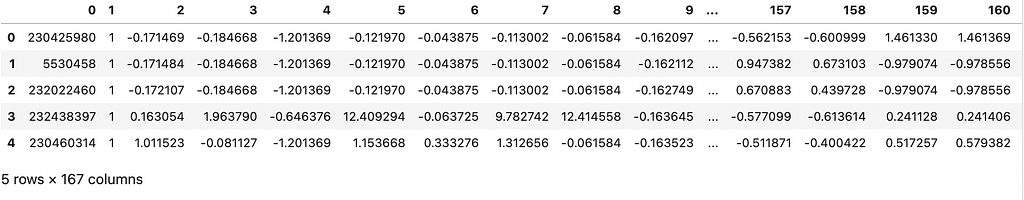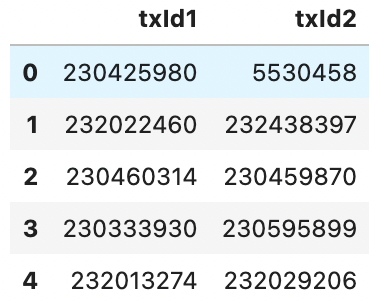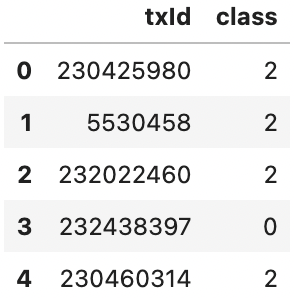0 — legitimate
1 — fraud
2 — unknown class

df_classes[‘class’].value_counts()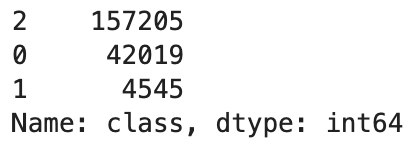Preparing edges

https://medium.com/media/8fc34dfcc566bf7613a13d8d7b0d7c42/href

Total amount of edges in DAG: torch.Size([2, 234355])

Preparing nodes

Let’s ignore the temporal axis and consider the static case of fraud detection.

https://medium.com/media/4ffb171b368d3fdc65809de5cc1f5e43/href

PyG Dataset

https://medium.com/media/ca8a6909418dd24e5e82fe29f8ebe27f/href

Number of nodes: 203769
Number of node features: 165
Number of edges: 234355
Number of edge features: 165
Average node degree: 1.15
Number of classes: 2
Has isolated nodes: False
Has self loops: False
Is directed: True

https://medium.com/media/dcf0ecbfcdc754b702917123e132d7c3/href

Train dataset size: 39579
Validation dataset size: 6985
Test dataset size: 157205

Models

https://medium.com/media/3526827c39b772a06a903562a149cb73/href

Train/test helpers

https://medium.com/media/37804423435e09fa8c8b5787b623570f/href

Train GCN

https://medium.com/media/f75cc1e070a905277c322d5893de22fe/href

Epoch 0 | Train Loss: 0.759 | Train Acc: 62.16% | Val Loss: 0.73 | Val Acc: 64.07%
Saving model for best loss
Epoch 10 | Train Loss: 0.307 | Train Acc: 86.43% | Val Loss: 0.30 | Val Acc: 87.16%
Saving model for best loss
Epoch 20 | Train Loss: 0.258 | Train Acc: 89.52% | Val Loss: 0.25 | Val Acc: 89.61%
Saving model for best loss
Epoch 30 | Train Loss: 0.244 | Train Acc: 90.49% | Val Loss: 0.24 | Val Acc: 90.32%
Saving model for best loss
Epoch 40 | Train Loss: 0.230 | Train Acc: 91.32% | Val Loss: 0.22 | Val Acc: 91.40%
Saving model for best loss
Epoch 50 | Train Loss: 0.219 | Train Acc: 91.85% | Val Loss: 0.22 | Val Acc: 91.77%
Saving model for best loss
Epoch 60 | Train Loss: 0.214 | Train Acc: 92.35% | Val Loss: 0.21 | Val Acc: 92.61%
Saving model for best loss
Epoch 70 | Train Loss: 0.210 | Train Acc: 92.60% | Val Loss: 0.21 | Val Acc: 92.80%
Saving model for best loss
Epoch 80 | Train Loss: 0.201 | Train Acc: 92.86% | Val Loss: 0.20 | Val Acc: 92.81%
Saving model for best loss
Epoch 90 | Train Loss: 0.195 | Train Acc: 93.15% | Val Loss: 0.20 | Val Acc: 92.81%
Saving model for best loss
Epoch 100 | Train Loss: 0.194 | Train Acc: 93.25% | Val Loss: 0.19 | Val Acc: 93.53%
Saving model for best loss

Test GCN

https://medium.com/media/92c354e11ee4b0f2980789bda7869dc8/href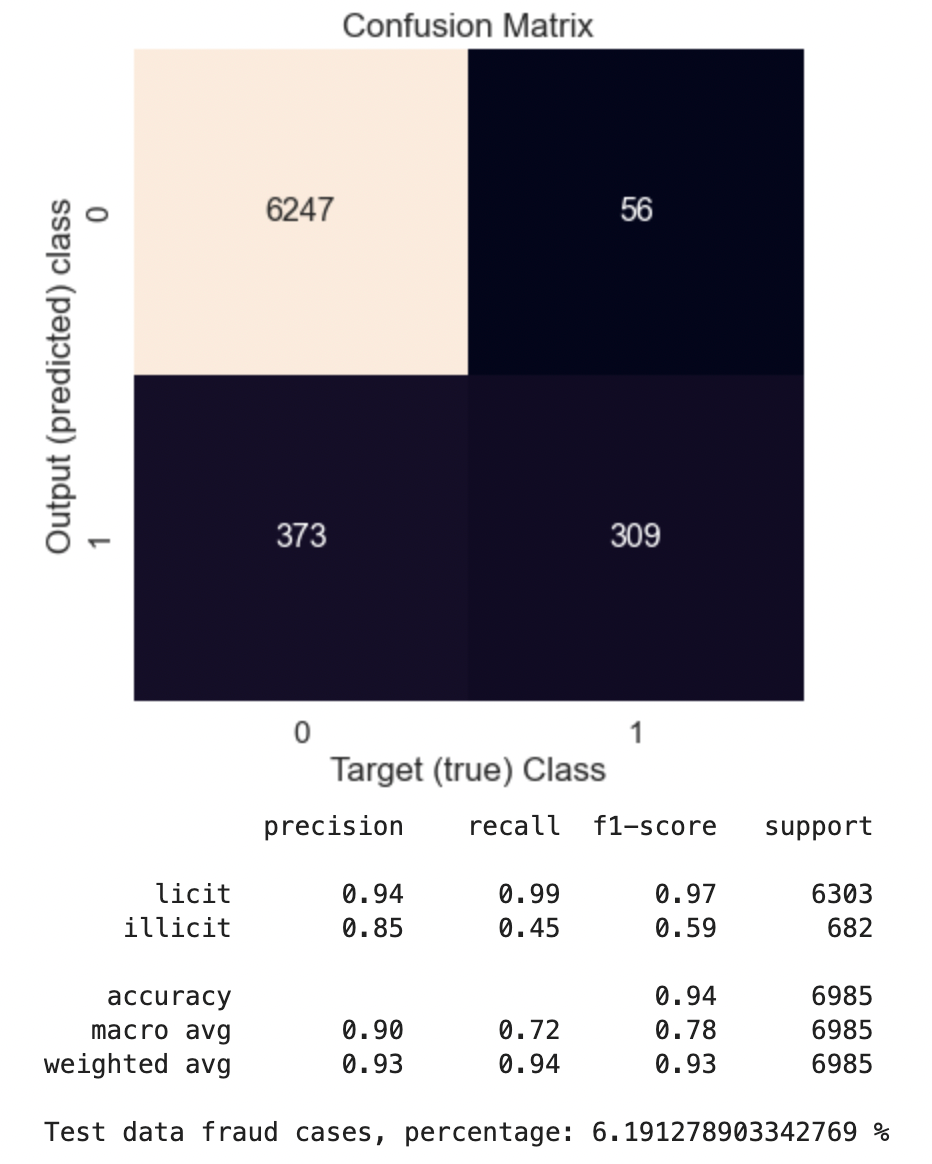Train GAT

https://medium.com/media/1a9cc229f1c3b3c89e948b403d388bf8/href

Epoch 0 | Train Loss: 1.176 | Train Acc: 68.34% | Val Loss: 1.01 | Val Acc: 68.33%
Saving model for best loss
Epoch 10 | Train Loss: 0.509 | Train Acc: 88.63% | Val Loss: 0.48 | Val Acc: 88.70%
Saving model for best loss
Epoch 20 | Train Loss: 0.489 | Train Acc: 90.09% | Val Loss: 0.49 | Val Acc: 89.94%
Epoch 30 | Train Loss: 0.465 | Train Acc: 89.87% | Val Loss: 0.48 | Val Acc: 89.76%
Saving model for best loss
Epoch 40 | Train Loss: 0.448 | Train Acc: 89.81% | Val Loss: 0.44 | Val Acc: 90.15%
Saving model for best loss
Epoch 50 | Train Loss: 0.445 | Train Acc: 90.04% | Val Loss: 0.44 | Val Acc: 89.89%
Epoch 60 | Train Loss: 0.443 | Train Acc: 90.22% | Val Loss: 0.44 | Val Acc: 90.45%
Epoch 70 | Train Loss: 0.439 | Train Acc: 90.38% | Val Loss: 0.43 | Val Acc: 90.16%
Saving model for best loss
Epoch 80 | Train Loss: 0.426 | Train Acc: 90.57% | Val Loss: 0.43 | Val Acc: 90.41%
Saving model for best loss
Epoch 90 | Train Loss: 0.423 | Train Acc: 90.72% | Val Loss: 0.42 | Val Acc: 90.38%
Saving model for best loss
Epoch 100 | Train Loss: 0.418 | Train Acc: 90.72% | Val Loss: 0.42 | Val Acc: 90.74%
Saving model for best loss

Test GAT

https://medium.com/media/8a6ebe40f40473e93e2bbdaf4109d012/href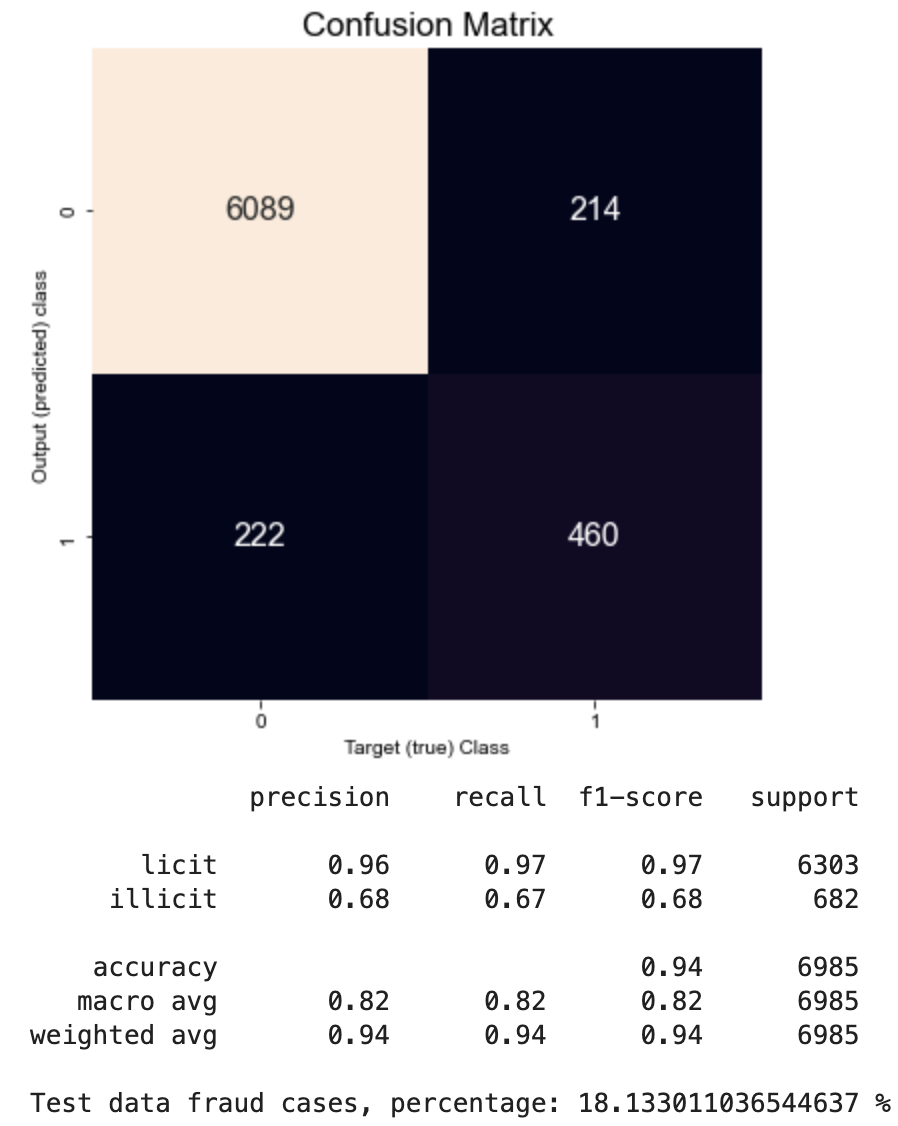👉🏻 Full code is also accessible through my GitHub.

Conclusion

From the calculation results, we can see that the GAT model converges slower in comparison to GCN, and train/validation accuracies are slightly lower than GCN. However, the confusion matrix built from validation data (labeled data) shows that the recall metric improved from 0.45 (GCN) to 0.67 (GAT). Thus, the GAT model more clearly identifies fraudsters than GCN but is also more strict with licit cases. Tests on unlabelled data containing 157205 samples show that in the case of GCN, there are only 6 % of fraud cases, while in the case of GAT, this amount is about 18 %.

#### References

Bronstein M. et al., Geometric deep learning: going beyond Euclidean data (2017), IEEE SIG PROC MAG, https://arxiv.org/pdf/1611.08097.pdf
Kipf T. N., Welling M. Semi-supervised classification with graph convolutional networks (2017), ICLR, https://arxiv.org/pdf/1609.02907.pdf
Zhou J. et al., Graph neural networks: A review of methods and applications (2020), AI Open, Volume 1, https://doi.org/10.1016/j.aiopen.2021.01.001
Velickovic P. et al., Graph Attention Networks (2018), ICLR, https://arxiv.org/pdf/1710.10903.pdfGraph Neural Networks for Fraud Detection in Crypto Transactions was originally published in Towards AI on Medium, where people are continuing the conversation by highlighting and responding to this story.

Published via Towards AI